Filling small holes

A MATLAB user recently asked in the MATLAB newsgroup how to fill "small" holes in a binary image. The function imfill can be used to fill all holes, but this user only wanted to fill holes having an area smaller than some threshold.

That's an interesting question. It can be done using a combination of imfill, bwareaopen, and MATLAB logical operators. Here's how.

Step 1: Fill all holes using imfill:

imshow(original)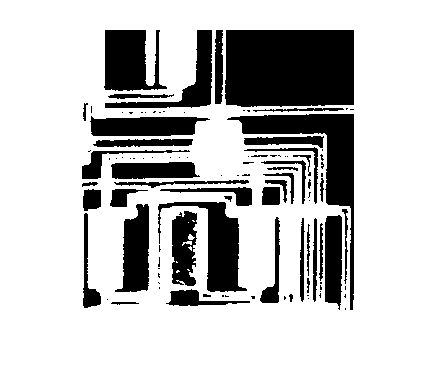filled = imfill(original, 'holes');
imshow(filled)
title('All holes filled')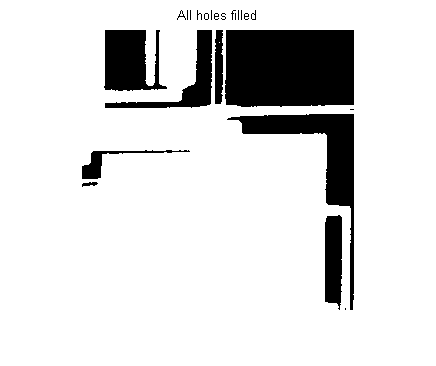Step 2: Identify the hole pixels using logical operators:

holes = filled & ~original;
imshow(holes)
title('Hole pixels identified')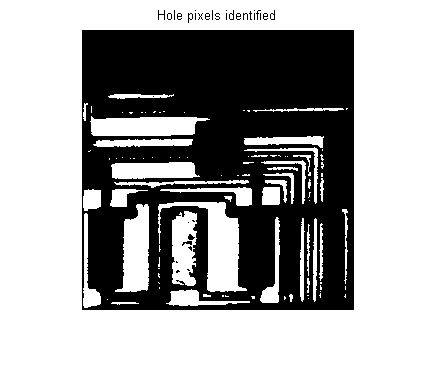Step 3: Use bwareaopen on the holes image to eliminate small holes:

bigholes = bwareaopen(holes, 200);
imshow(bigholes)
title('Only the big holes')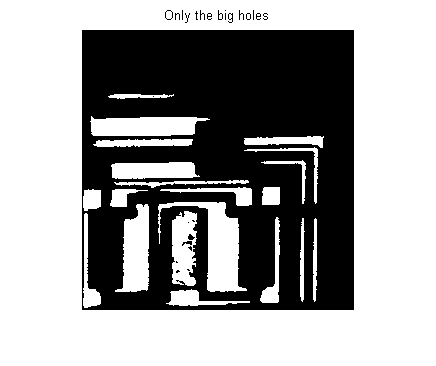Step 4: Use logical operators to identify small holes:

smallholes = holes & ~bigholes;
imshow(smallholes)
title('Only the small holes')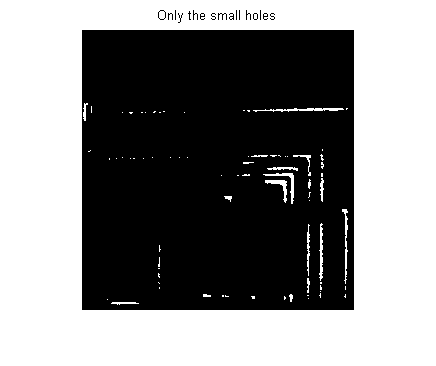Step 5: Use a logical operator to fill in the small holes in the original image:

new = original | smallholes;
imshow(new)
title('Small holes filled')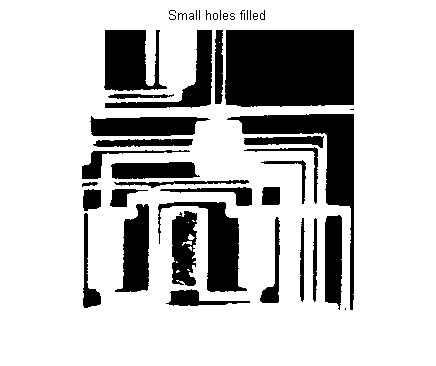All done!

Published with MATLAB® 7.6

|